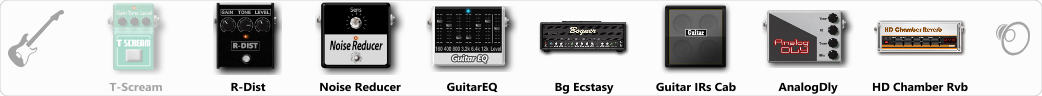Discussion in 'ToneLib-GFX presets' started by Gaborock, Aug 23, 2019.

Effects chain:Effect: "T-Scream" (Overdrive / Distortion), active - "no"
"Drive" = 22
"Tone" = 80
"Level" = 79

Effect: "R-Dist" (Overdrive / Distortion), active - "yes"
"Drive" = 47
"Tone" = 80
"Level" = 42

Effect: "Noise Reducer" (Dynamics / Filter), active - "yes"
"Sens" = 50
"Mode" = Hard

Effect: "GuitarEQ" (Dynamics / Filter), active - "yes"
"160 Hz" = 0
"400 Hz" = 0
"800 Hz" = -5
"1.6 kHz" = 0
"3.2 kHz" = 0
"6.4 kHz" = 0
"12 kHz" = 0
"Level (dB)" = 0

Effect: "Bg Ecstasy" (Amp simulators), active - "yes"
"Gain" = 50
"Bass" = 50
"Middle" = 50
"Treble" = 50
"Presence" = 50
"Master" = 50
"Output" = 50
"Level (dB)" = 0

Effect: "Guitar IRs Cab" (Cabinets), active - "yes"
"Model" = Fender Deluxe Rev (1x12")
"Mic Position" = Middle
"Mic Distance" = Near
"Low Cut (Hz)" = 60
"Hi Cut (kHz)" = 20.0
"Mix" = 100
"Level (dB)" = 0

Effect: "AnalogDly" (Delay), active - "yes"
"Time" = 380
"Feedback" = 26
"Tone" = 65
"Mix" = 60

Effect: "HD Chamber Rvb" (Reverb), active - "yes"
"Time" = 8.2
"PreLPF" = 79
"PreDelay" = 388
"HiDamp" = 21
"LoGain" = -2.1
"Mix" = 37

Note: You will need to download and install the ToneLib-GFX software to use the preset.